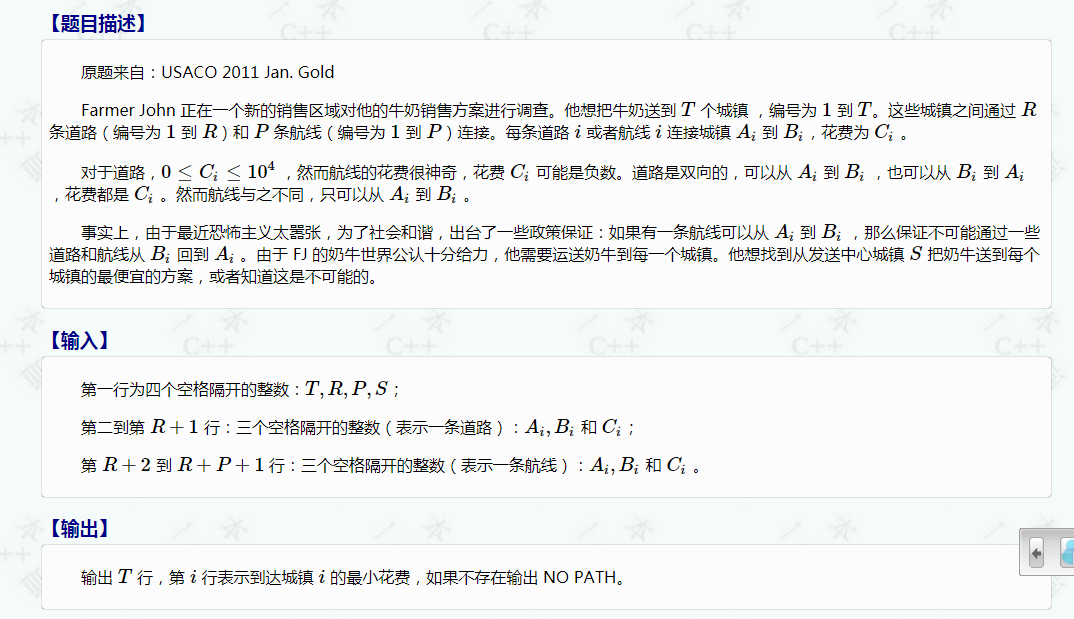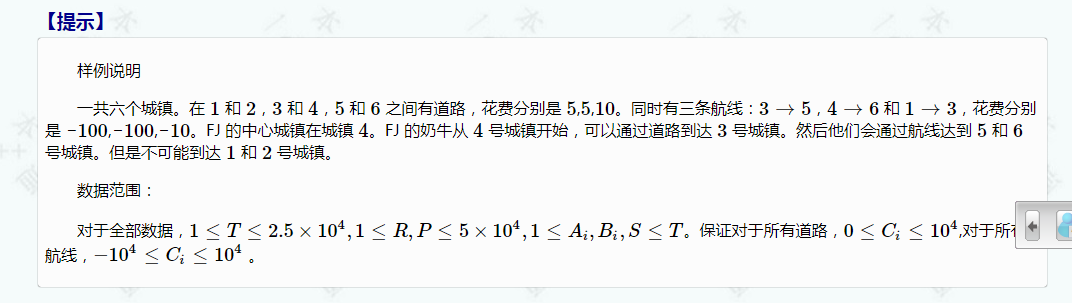Farmer John is conducting research for a new milk contract in a new territory. He intends to distribute milk to T (1 <= T <= 25,000) towns conveniently numbered 1..T which are connected by up to R (1 <= R <= 50,000) roads conveniently numbered 1..R and/or P (1 <= P <= 50,000) airplane flights conveniently numbered 1..P.

Either road i or plane i connects town A_i (1 <= A_i <= T) to town B_i (1 <= B_i <= T) with traversal cost C_i. For roads, 0 <= C_i <= 10,000; however, due to the strange finances of the airlines, the cost for planes can be quite negative (-10,000 <= C_i <= 10,000).

Roads are bidirectional and can be traversed from A_i to B_i or B_i to A_i for the same cost; the cost of a road must be non-negative.

Planes, however, can only be used in the direction from A_i to B_i specified in the input. In fact, if there is a plane from A_i to B_i it is guaranteed that there is no way to return from B_i to A_i with any sequence of roads and planes due to recent antiterror regulation.

Farmer John is known around the world as the source of the world's finest dairy cows. He has in fact received orders for his cows from every single town. He therefore wants to find the cheapest price for delivery to each town from his distribution center in town S (1 <= S <= T) or to know that it is not possible if this is the case.

MEMORY LIMIT: 64MB

IN

• Line 1: Four space separated integers: T, R, P, and S
• Lines 2..R+1: Three space separated integers describing a road: A_i, B_i and C_i
• Lines R+2..R+P+1: Three space separated integers describing a plane: A_i, B_i and C_i

OUT

• Lines 1..T: The minimum cost to get from town S to town i, or 'NO PATH' if this is not possible#include <iostream>
#include <deque>
#include <cstring>
#define M 192000
#define N 51200

using namespace std;

struct Edge
{
int to,dis,next;
}    edge[M];
int head[N],deg;

inline void add(int from,int to,int dis)
{
edge[++deg].next=head[from];
edge[deg].dis=dis;
edge[deg].to=to;
head[from]=deg;
}

int n,R,P,s;

inline void init()
{
cin.tie(0);
cin>>n>>R>>P>>s;
for(int i=1;i<=R;i++)
{
int f,t,d;
cin>>f>>t>>d;
add(f,t,d);add(t,f,d);
}
for(int i=1;i<=P;i++)
{
int f,t,d;
cin>>f>>t>>d;
add(f,t,d);
}
}

int dist[N],ved[N];

inline void spfa(int from)    //spfa&SLF
{

deque<int> Q;
memset(dist,0x3f,sizeof(dist));
memset(ved,0,sizeof(ved));

Q.push_front(from);
dist[from]=0;
ved[from]=true;

while(!Q.empty())
{
int k=Q.front();
Q.pop_front();

ved[k]=false;

for(int i=head[k];i!=0;i=edge[i].next)
if(dist[edge[i].to]>dist[k]+edge[i].dis)
{
dist[edge[i].to]=dist[k]+edge[i].dis;
if(!ved[edge[i].to])
{
if(!Q.empty() && dist[edge[i].to]<dist[Q.front()])
Q.push_front(edge[i].to);
else
Q.push_back(edge[i].to);
ved[edge[i].to]=true;
}
}
}

for(int i=1;i<=n;i++)
{
if(dist[i]!=0x3f3f3f3f)
cout<<dist[i]<<endl;
else
cout<<"NO PATH"<<endl;
}
}

int main()
{
init();
spfa(s);
}

Last modification：February 7th, 2021 at 04:30 pm